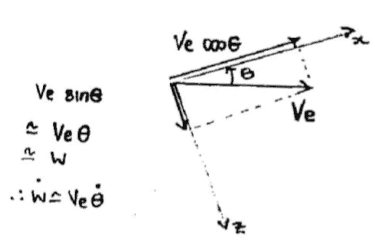# Why is this true about the angle theta

## Homework Statement

From my notes:Why is:
$$V_{e} \times sin \Theta \cong V_{e}\Theta$$ ?

The only thing I know is that the assumption: $$\Theta \rightarrow 0$$ has been made, but that doesn't make the above equation clear to me...

I'm not 100% sure what you're asking, but it looks like you are just talking about the small angle approximation of the sine function. That's easy to show if you know the Taylor Expansion for sine.

$$\sin{\theta} = \theta - {\theta^3 \over 3!} + {\theta^5 \over 5!} - ...$$

Now, since $$\theta$$ is very small, any positive integer power of $$\theta$$ will go to 0 even faster than $$\theta$$ itself. ie. $$\theta^3 \to 0$$, $$\theta^5 \to 0$$, etc.

So $$\sin{\theta} \approx \theta$$

That was exactly my question, the answer is clear, thanks a lot!